Computational Seismology
Finite Differences - Taylor Operators and Numerical DifferentiationThis notebook is part of the supplementary material to Computational Seismology: A Practical Introduction, Oxford University Press, 2016.

##### Authors:¶

This exercise covers the following aspects:

• Use the Taylor series to calculate finite-difference operators
• Learn how to define high-order finite-difference operators
• Demonstrate the accuracy improvement by testing the operators on (arbitrary) functions

## Basic Equations¶

The Taylor expansion of $f(x + dx)$ around $x$ is defined as

$$f(x+dx)=\sum_{n=0}^\infty \frac{f^{(n)}(x)}{n!}dx^{n}$$

Finite-difference operators can be calculated by seeking weights (here: $a$, $b$, $c$) with which function values have to be multiplied to obtain an interpolation or a derivative. Example:

\begin{align} a ~ f(x + dx) & \ = \ a ~ \left[ ~ f(x) + f^{'} (x) dx + \frac{1}{2!} f^{''} (x) dx^2 + \dotsc ~ \right] \\ b ~ f(x) & \ = \ b ~ \left[ ~ f(x) ~ \right] \\ c ~ f(x - dx) & \ = \ c ~ \left[ ~ f(x) - f^{'} (x) dx + \frac{1}{2!} f^{''} (x) dx^2 - \dotsc ~ \right] \end{align}

This can be expressed in matrix form by comparing coefficients, here seeking a 2nd derivative

\begin{align} &a ~~+~~ ~~~~b &+~~ c & = & 0 \\ &a ~~\phantom{+}~~ \phantom{b} &-~~ c & = & 0 \\ &a ~~\phantom{+}~~ \phantom{b} &+~~ c & = & \frac{2!}{\mathrm{d}x^2} \end{align}

$$\begin{pmatrix} 1 & 1 & 1 \\ 1 & 0 & -1 \\ 1 & 0 & 1 \end{pmatrix} \begin{pmatrix} a\\ b \\ c \end{pmatrix} = \begin{pmatrix} 0\\ 0 \\ \frac{2!}{dx^2} \end{pmatrix}$$

and using matix inversion we obtain

$$\begin{pmatrix} a \\ b\\ c \end{pmatrix} = \begin{pmatrix} \frac{1}{2 \mathrm{d}x^2} \\ - \frac{2}{2 \mathrm{d}x^2} \\ \frac{1}{2 \mathrm{d}x^2} \end{pmatrix}$$

This is the the well known 3-point operator for the 2nd derivative. This can easily be generalized to higher point operators and higher order derivatives. Below you will find a routine that initializes the system matrix and solves for the Taylor operator.

## Calculating the Coefficients¶

The subroutine central_difference_coefficients() initalizes the system matrix and solves for the difference weights assuming $dx=1$. It calculates the centered differences using an arbitrary number of coefficients, also for higher derivatives.

It returns a central finite difference stencil of order points for the nth derivative.

In :
%matplotlib inline

import math
import numpy as np

def central_difference_coefficients(points, n):
"""
Calculate the central finite difference stencil for an arbitrary number
of points and an arbitrary order derivative.

:param points: The number of points for the stencil. Must be
an odd number.
:param n: The derivative order. Must be a positive number.
"""
if not points % 2:
raise ValueError("Only uneven number of points allowed.")
if points <= n:
raise ValueError("The %ith derivative requires at least %i points" %
(n, n + 2))
a = points
m = np.zeros((a, a))
for i in range(a):
for j in range(a):
dx = j - a // 2
m[i][j] = dx ** i

s = np.zeros(a)
s[n] = math.factorial(n)
return np.linalg.solve(m, s)


## (Almost) Christmas¶

The coefficients quickly decay and yield diminishing returns for higher order stencils. Higher order derivatives naturally require longer stencils.

In :
import matplotlib.pyplot as plt
plt.style.use('ggplot')

plt.figure(figsize=(10, 8))

for _i, points in enumerate(range(3, 20, 2)):
coeffs = central_difference_coefficients(points, 1)
numbers = np.linspace(- (points // 2), points // 2, points)
plt.plot(numbers, coeffs - _i * 1, color="#55A868", lw=2.5)
plt.plot(numbers, coeffs - _i * 1, color="#F1DB40", lw=0, marker="*", markersize=15)
plt.hlines(-(_i * 1), -100, 100, color="0.5", zorder=0)

plt.scatter(, [1.5], marker="*", s=1000, color="#C95241")

plt.yticks([])
plt.vlines(0, *plt.ylim(), color="0.5", zorder=0)
plt.xlim(-(points // 2 + 1), points // 2 + 1)
plt.ylim(-(_i + 2), 3)
plt.title("FD Tree for the first derivative")
plt.show()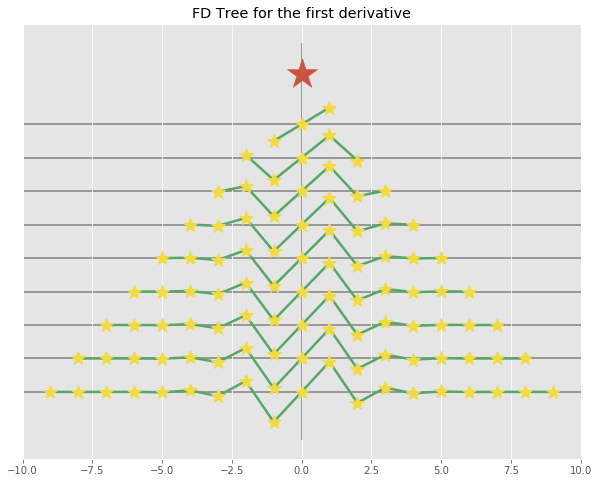In :
import matplotlib.pyplot as plt
plt.style.use('ggplot')

plt.figure(figsize=(10, 8))

for _i, points in enumerate(range(3, 20, 2)):
coeffs = central_difference_coefficients(points, 2)
numbers = np.linspace(- (points // 2), points // 2, points)
plt.plot(numbers, coeffs - _i * 3, color="#55A868", lw=2.5)
plt.plot(numbers, coeffs - _i * 3, color="#F1DB40", lw=0, marker="*", markersize=15)
plt.hlines(-(_i * 3), -100, 100, color="0.5", zorder=0)

plt.scatter(, [3.5], marker="*", s=1000, color="#C95241")

plt.yticks([])
plt.vlines(0, *plt.ylim(), color="0.5", zorder=0)
plt.xlim(-(points // 2 + 1), points // 2 + 1)
plt.ylim(-(3 * _i + 2 * 3), 6)
plt.title("FD Tree for the second derivative")
plt.show()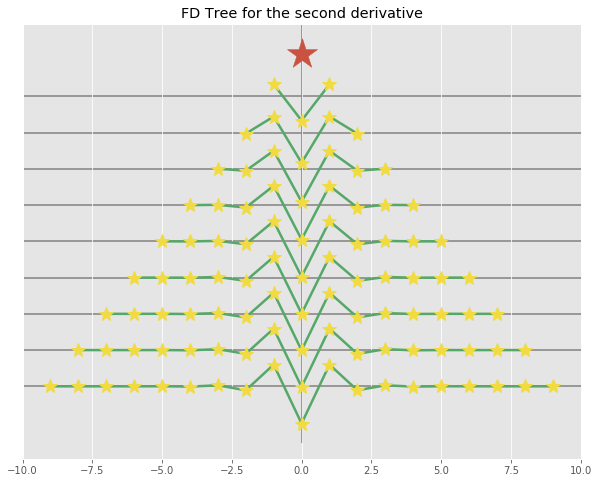In :
import matplotlib.pyplot as plt
plt.style.use('ggplot')

plt.figure(figsize=(10, 8))

for _i, points in enumerate(range(5, 20, 2)):
coeffs = central_difference_coefficients(points, 3)
numbers = np.linspace(- (points // 2), points // 2, points)
plt.plot(numbers, coeffs - _i * 3, color="#55A868", lw=2.5)
plt.plot(numbers, coeffs - _i * 3, color="#F1DB40", lw=0, marker="*", markersize=15)
plt.hlines(-(_i * 3), -100, 100, color="0.5", zorder=0)

plt.scatter(, [5.5], marker="*", s=1000, color="#C95241")

plt.yticks([])
plt.vlines(0, *plt.ylim(), color="0.5", zorder=0)
plt.xlim(-(points // 2 + 1), points // 2 + 1)
plt.ylim(-(3 * _i + 2 * 3), 7)
plt.title("FD Tree for the third derivative")
plt.show()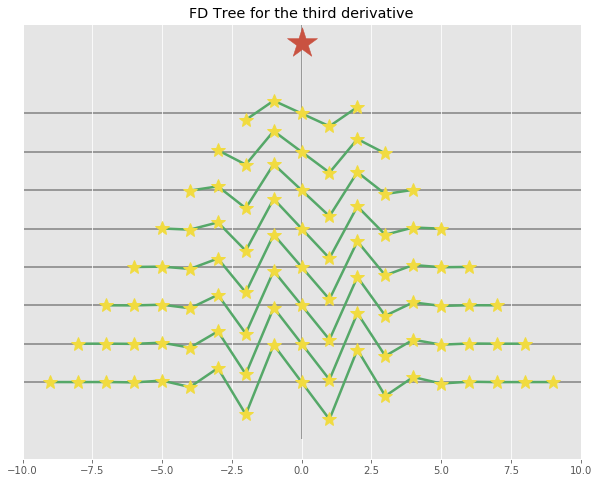## Accuracy of centered finite differences¶

Define a simple function, e.g. sine, cosine, gaussian, some polynomial, ..., differentiate it using the stencils returned from the above function and compare the result to the analytically calculated derivative.

In :
sine_curve = np.sin(np.linspace(0, 10 * np.pi, 10000))
dx = 10 * np.pi / 10000

plt.figure(figsize=(12, 6))
plt.subplot(211)
plt.plot(sine_curve)

stencil = central_difference_coefficients(5, 1)

# Dummy application.
cosine_curve = np.zeros(len(sine_curve))
for _i in range(2, len(sine_curve) - 2):
cosine_curve[_i] = stencil * sine_curve[_i - 2] + stencil * sine_curve[_i - 1] + \
stencil * sine_curve[_i] + stencil * sine_curve[_i + 1] + \
stencil * sine_curve[_i + 2]
# Scale by dt.
cosine_curve /= dx

plt.subplot(212)
plt.plot(cosine_curve[10:-10], label="Numerical derivative", lw=2)
plt.plot(np.cos(np.linspace(0, 10 * np.pi, 10000))[10:-10], ls="--",
label="Analytic derivative")
plt.ylim(-1.1, 1.1)
plt.xlim(0, 9980)
plt.legend()
plt.show()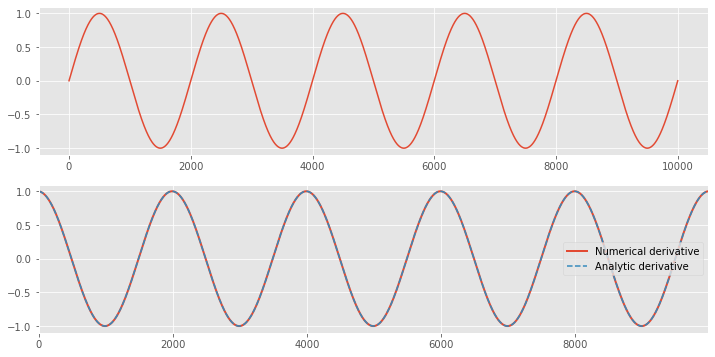### Exercise 2¶

This exercise aims to illustrate the accuracy gains by using longer finite difference stencils. To this end we define a helper function that takes an array of x values, an array of the function sampled at these values to differentiate, the analytical derivative, the length of the stencil, and the order of the derivative.

In :
def diff_helper(x, data, analytical_diff, stencil_length, diff_order):
plt.close()
dx = x - x

plt.figure(figsize=(12, 5))
plt.subplot(311)
# Be super conservative about edge effect.
buf = stencil_length // 2 + 1
x = x[buf:-buf]
plt.plot(x, data[buf:-buf], label="Function")
plt.legend()

stencil = central_difference_coefficients(stencil_length, diff_order)

# Its effectively a convolution. So use a pre-built convolution function.
diff = np.convolve(data, stencil[::-1])
diff /= dx ** diff_order
diff = diff[stencil_length // 2: -(stencil_length // 2)]

plt.subplot(312)
plt.plot(x, diff[buf:-buf], label="Numerical derivative", lw=2)
plt.plot(x, analytical_diff[buf:-buf], ls="--", label="Analytic derivative")
plt.legend()

plt.subplot(313)
plt.plot(x, (diff - analytical_diff)[buf:-buf], label="Numerical - Analytical Derivative")
plt.legend()

plt.tight_layout()
plt.show()


This shows a simple usage example. Play around a bit to get a feel how the accuracy improves with higher order operators. Make sure the function is not too oversampled, otherwise the effect is naturally not very strong. Polynomials of degree $N-1$ are exactly differentiated (first derivatives) by central differences of order $\ge N$. The coefficients are derived from Taylor polynomials after all.

In :
x = np.linspace(-2, 2, 150)
p = np.poly1d([1, 0, -2, 1, 0])
p_diff = np.poly1d([4, 0, -4, 1])
diff_helper(x, p(x), p_diff(x), 3, 1)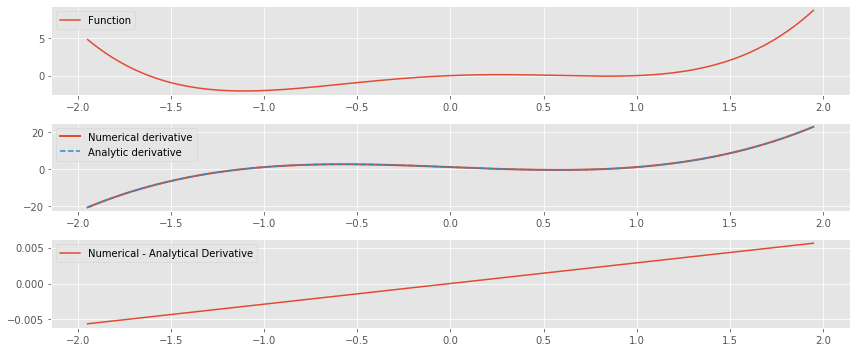## Bonus: Theoretical Accuray¶

In :
def H(N, omega):
coeffs = central_difference_coefficients(N, 1)
HH = np.zeros_like(omega)
for _i, c in enumerate(coeffs):
HH += c * np.sin((-(N // 2) + _i) * omega)
return HH

omega = np.linspace(0.0, np.pi, 100)

f = omega / (2 * np.pi)

plt.plot(f * 200, omega, color='0.2', ls="--")
for _i in range(3, 17, 2):
plt.plot(f * 200, H(_i, omega), label="%i points" % _i)
plt.legend(loc="upper left")
plt.xlabel("% Nyquist")
plt.ylabel("$|H(\omega)|$")
plt.title("Amplitude Response of First Derivative Stencils")
plt.show()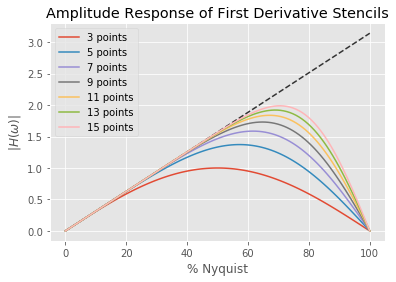In :
# Some tests against analytically calculated stencils. Not important for the exercises.

# f'(x) 5-point-stencil.
np.testing.assert_allclose(
central_difference_coefficients(5, 1),
np.array([-1.0 / 12.0, + 2.0 / 3.0, 0, -2.0 / 3.0, 1.0 / 12.0])[::-1],
rtol=1E-8, atol=1E-10)

# f''(x) 5-point-stencil.
np.testing.assert_allclose(
central_difference_coefficients(5, 2),
np.array([-1.0 / 12.0, + 16.0 / 12.0, -30.0 / 12.0, 16.0 / 12.0, -1.0 / 12.0])[::-1],
rtol=1E-8, atol=1E-10)

# f'''(x) 5-point-stencil.
np.testing.assert_allclose(
central_difference_coefficients(5, 3),
np.array([1.0 / 2.0, -1.0, 0, 1.0, -1.0 / 2.0])[::-1],
rtol=1E-8, atol=1E-10)

# f''''(x) 5-point-stencil.
np.testing.assert_allclose(
central_difference_coefficients(5, 4),
np.array([1.0, -4.0, 6.0, -4.0, 1.0])[::-1],
rtol=1E-8, atol=1E-10)